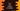# C++ program to find the volume of a cylinder in 3 different ways## How to find the volume of a cylinder:

In this post, we will learn learn how to find the volume of a cylinder with user input values. The formula we need to find the cylinder volume is πr^2h, where r is the radius of the cylinder, h is the height of the cylinder, and π is the mathematical constant PI.

Since π is a constant value, we need the radius and height of a cylinder to calculate its volume. The program will take these values as inputs from the user, calculate the volume and print it out.

### Method 1: C++ program to find the volume of a cylinder:

The below program calculates the volume of a cylinder:

``````#include <iostream>
using namespace std;

int main()
{
double PI = 3.14159;

cout << "Enter the height of the cylinder: " << endl;
cin >> height;

cout << "Enter the radius of the cylinder: " << endl;

cout << "The volume of the cylinder is: " << volume << endl;
}``````

Here,

• The height, radius and volume are three double variables to hold the height, radius and volume of the cylinder.
• PI is a double value to hold the value of π.
• It asks the user to enter the height and radius of the cylinder. It reads the user input values and assigns these to the height and radius variables.
• The calculated volume value is assigned to the volume variable and the last line prints its value.

If you run this program, it will print output as like below:

``````Enter the height of the cylinder:
33
Enter the radius of the cylinder:
5
The volume of the cylinder is: 2591.81

Enter the height of the cylinder:
32
Enter the radius of the cylinder:
22
The volume of the cylinder is: 48656.9``````

### Method 2: C++ program to find the volume of a cylinder using predefined PI value:

In the above example, we used the π value from a predefined constant. It’s value is already defined in the cmath header file. Let’s learn how to use this value from cmath in the below example:

``````#include <iostream>
#include <cmath>
using namespace std;

int main()
{

cout << "Enter the height of the cylinder: " << endl;
cin >> height;

cout << "Enter the radius of the cylinder: " << endl;

cout << "The volume of the cylinder is: " << volume << endl;
}``````

As you can see here,

• cmath header is included to the start of the program.
• M_PI is the constant that holds the PI value. We are using it to calculate the volume.

Sample output:

``````Enter the height of the cylinder:
100
Enter the radius of the cylinder:
22
The volume of the cylinder is: 152053``````

### Method 3: C++ program to find the volume of a cylinder using a separate function:

Let’s use a separate function to calculate the volume of the cylinder. This method will be called from main() or any other method and it will calculate and return the volume.

``````#include <iostream>
#include <cmath>
using namespace std;

{
}

int main()
{

cout << "Enter the height of the cylinder: " << endl;
cin >> height;

cout << "Enter the radius of the cylinder: " << endl;